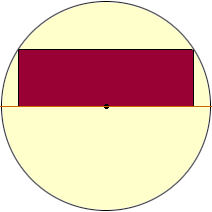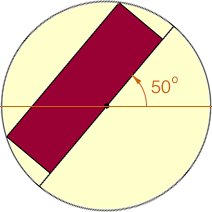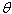SEARCH HOMEMath Central Quandaries & QueriesQuestion from Graham: There is a circle inside which from the centre to the top is a rectangle. Size is unimportant. If I the rectangle is at 0 degrees and I know the co-ordinates of the 4 corners I can do a check to see whether a given point x,y is inside the rectangle or not. Question : If the rectangle is rotated by 50 degrees how do I check then as the lines of the rectangle are not perfectly straight with regards to x and y ie at 0 degrees x changes but y is constant and vice versa. At 50 degrees x and y change together. Many thanks GrahamHi Graham,

Angles are usually measured in a counterclockwise directionso I am going to assume that the rectangle is rotated 50 degrees counterclockwise around the centre of the circle wich is also the origin. Here are my diagrams of the rectangle and the rotated rectangle.original positionrotated 50o

You now have a point (x, y) in the plane and you want to check to see if it is inside the rotated rectangle. I would take the point (x, y), rotate it 50 degrees clockwise around the centre of the circle and then check to see if the resulting point is inside the original rectangle.

Suppose that you have a point (x, y) in the plane and and you want to rotate itdegrees counterclockwise around the origin to arrive at a new point (x', y') then the relationship between (x, y) and (x', y') is

x' = x cos() - y sin()
y' = y cos() + x sin()

In your situation you want to rotate the point 50o clockwise so= - 50o. Start with the point in question, (x, y), transform it to (x', y') by

x' = x cos(-50o) - y sin(-50o)
y' = y cos(-50o) + x sin(-50o)

and check to see if (x', y') is in the original rectangle.

PennyMath Central is supported by the University of Regina and The Pacific Institute for the Mathematical Sciences.